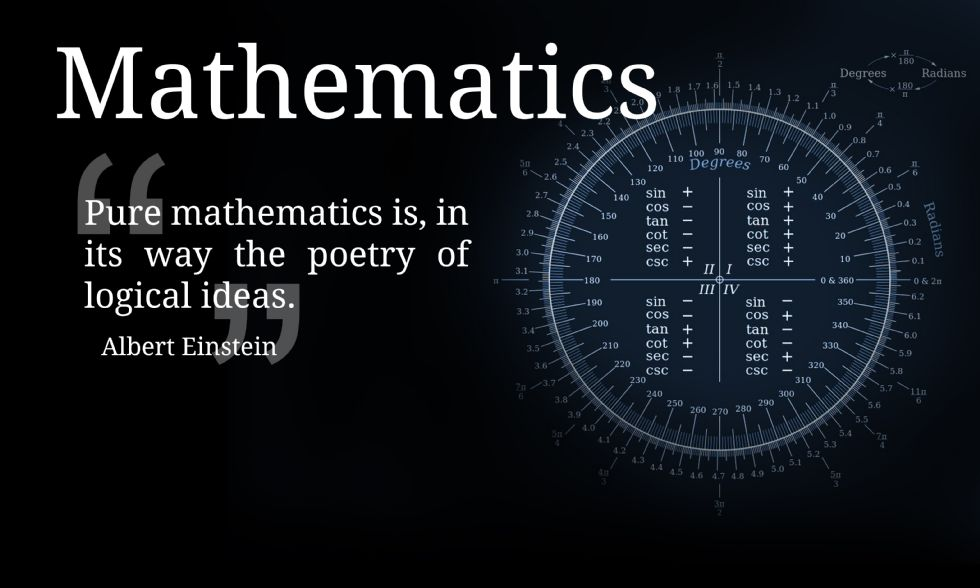Water Orton Road, Castle Bromwich, Birmingham. B36 9HF

Telephone:0121 748 0400

Fax:0121 748 0414

Contact:Mr Cox

# Mathematics## Head of Department - Mr Plackowski

Mathematics is a core subject in both Key Stage 3 and 4. Pupils will have 8 hours of Mathematics lesson per fortnight. Every pupil will study GCSE Mathematics in Key Stage 4, subject teachers carefully consider whether they will be entered for the higher or the foundation tier. Those pupils with a particular interest in the subject can also opt for Additional Maths and Statistics in Key Stage 4.

## Year 7

Assessment Cycle Content Covered
1

Sequences

Understand and use algebraic notation

Equality and equivalence

Place value and ordering integers and decimals

2

Fraction, decimal and percentage equivalence

Solving problems involving four operations

Fractions and percentages of amounts

Operations and equations with directed numbers

3

Constructing, measuring and using geometric notation

Developing geometric reasoning

4

Developing number sense

Sets and probability

Prime numbers and proof

## Year 8

Assessment Cycle Content Covered
1

Proportional reasoning

Multiplicative Change

Multiplying and Dividing Fractions

Working in the Cartesian Plane

2

Representing Data

Probability

Brackets, Equations and Inequalities

Sequences

Indices

3

Fractions and Percentages

Standard Form

Number Sense

Angles in Parallel Lines & Polygons

4

Area of Trapezia and Circles

Line Symmetry & Reflection

Data Handling Cycle

Measures of Location

## Year 9

Assessment Cycle Content Covered
1

Basic Calculation Skills

Powers and Roots

Approximation and Estimation

Operations with Fractions

2

Fractions, Decimals and Percentages

Collecting Like Terms

Expanding Brackets

Substitution

Solving Equations

3

Functions and Sequences

Properties of Shapes and Solids

Angles in Parallel Lines

Angles in Polygons

Perimeter and Area

Percentages

4

Ratio

Units and Measurements

Probability

## Year 10

Assessment Cycle Content Covered
1

Collecting and Displaying Data

Analysing Data

Probability

2

Probability

Units and Measurement

Perimeter and Area

Volume and Surface Area

Approximation and Estimation

3

Straight Line Graphs

Graphs of expressions and functions

Transformations

Pythagoras’ Theorem

Growth and Decay

4

Construction and Loci

Simultaneous Equations

## Year 11

Assessment Cycle Content Covered
1

Revision of Year 10 Content

Algebraic Expressions

Expanding Brackets

2

Analysing data

Functions and Sequences

Angles

Vector Geometry

Trigonometry

3 Revision and Exam Practice

## Year 10

Assessment Cycle Content Covered
1

Analysing data

Representing data

Probability

2

Units and Measurement

Perimeter and Area

Volume and Surface Area

Straight Line Graphs

Surds

3

Algebraic Fractions

Equations and Functions

Iteration

Simultaneous Equations

Algebraic Inequalities

4

Pythagoras’ Theorem

Trigonometry

## Year 11

Assessment Cycle Content Covered
1

Revision of Year 10 Content

Equations and Functions

Equations of Circles

2

Iteration

Vector calculations

Circle Theorems

Transformations and Graphs

Averages from Tables (Revision)

Collecting and displaying data (Revision)

3 Revision and Exam Practice

Additional maths is offered as an options course for pupils in Years 10 and 11.

## Year 10

Assessment Cycle Content Covered
1

Collecting data and sampling methods.

Representing and interpreting data.

2 Averages and measures of spread.
Surds.
Algebraic manipulation.
Polynomials, functions and equations.
Trigonometry.
3 Correlation including correlation coefficients.
Index numbers.
4

Time series graphs.

Moving averages.

## Year 11

Assessment Cycle Content Covered
1

Probability.
Standardised scores.

Exponentials and logarithms.

Numerical methods.

2

Differentiation.

Integration.

3 Kinematics.

Website Suggestions:

MathsWatch: www.mathswatchvle.com

BBC Bitesize KS3

BBC Bitesize KS4

Hegarty Maths (A-Level)

Maths Genie: www.mathsgenie.co.uk

Just Maths: www.justmaths.co.uk